## RS Aggarwal Solutions Class 10 Chapter 9 Mean, Median, Mode of Grouped Data,Cumulative Frequency Graph and O give Ex 9b

These Solutions are part of RS Aggarwal Solutions Class 10. Here we have given RS Aggarwal Solutions Class 10 Chapter 9 Mean, Median, Mode of Grouped Data,Cumulative Frequency Graph and O give Ex 9b.

Other Exercises

Question 1.
Solution:Question 2.
Solution:
We prepare the frequency table, given belowThe cumulative frequency is 25 and corresponding class is 21 – 28.
Thus, the median class is 21 – 28
l = 21, h = 7, f = 11, c = C.F. preceding class 21 – 28 is 14 and N/2 = 25Hence the median is 28.

Question 3.
Solution:
We prepare the frequency table given below: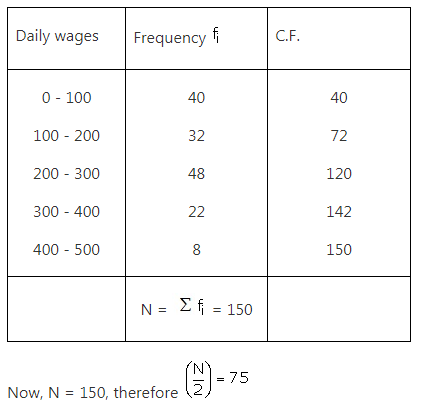The cumulative frequency just greater than 75 is 120 and corresponding class is 200 – 300.
Thus, the median class is 200 – 300
l = 200, h = 100, f = 48Hence the median of daily wages is Rs. 206.25.
Hence the median is 28.

Question 4.
Solution:
We prepare the frequency table, given below: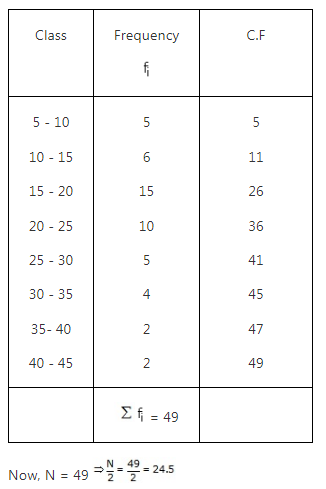The cumulative frequency just greater than 24.5 is 26 and corresponding class is 15 – 20.
Thus, the median class is 15 – 20
l = 15, h = 5, f = 15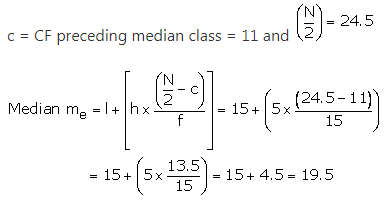Median of frequency distribution is 19.5.

Question 5.
Solution:
We prepare the cumulative frequency table as given below:The cumulative frequency just greater than 33.5 is 42 and the corresponding class 125 – 145.
Thus, the median class is 125 – 145
l = 125, h = 20, f= 20 and c = CF preceding the median class = 22, N/2 = 33.5.Hence median of electricity consumed is 136.5.

Question 6.
Solution:
Frequency table is given below: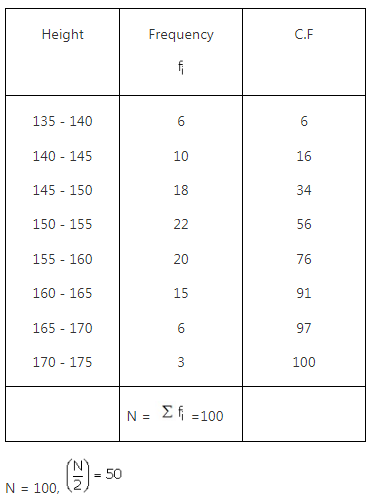The cumulative frequency just greater than 50 is 56 and the corresponding class is 150 – 155
Thus, the median class is 150 – 155
l = 150, h = 5, f = 22, c = C.F.preceding median class = 34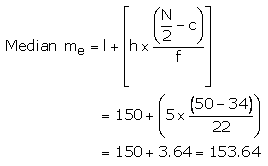Hence, Median = 153.64.

Question 7.
Solution:
The frequency table is given below. Let the missing frequency be x.l = 20, h = 10, f = x, c = C.F.
preceding median class = 30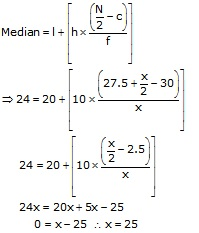Hence, the missing frequency is 25.

Question 8.
Solution:Question 9.
Solution:Question 10.
Solution:
Let f1 and fbe the frequencies of class intervals 0 – 10 and 40 – 50
f1+ 5 + 9 +12 + f2 + 3 + 2 = 40
⇒ f1+ f2 = 9
Median is 32.5 which lies in 30 – 40, so the median class is 30 – 40Question 11.
Solution:
The given series is of inclusive form. Converting it into exclusive form and preparing the cumulative frequency table, we get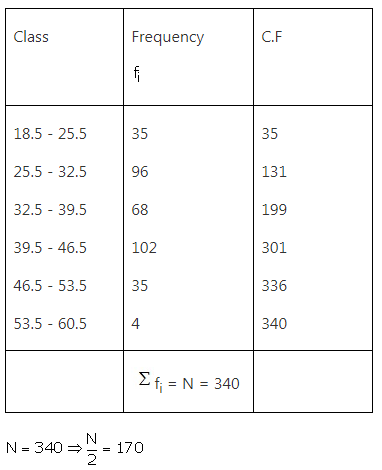The cumulative frequency just greater than 170 is 199 and the corresponding class is 32.5 – 39.5.
Median class is 32.5 – 39.5
l = 32.5, h = 7, f = 68, c = C.F. of preceding median class = 131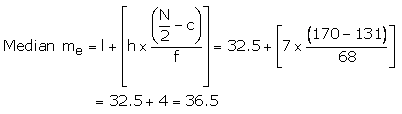Hence median is 36.5 years.

Question 12.
Solution:
Given series is in inclusive form converting it into exclusive form and preparing the cumulative frequency table, we getThe cumulative frequency just greater than 49 is 70 and corresponding class is 90.5 – 100.5.
median class is 90.5 – 100.5
l = 90.5, h = 10, f = 30, c = CF preceding median class = 40Hence, Median = Rs 93.50.

Question 13.
Solution:
The given series is converted from inclusive to exclusive form and preparing the cumulative frequency table, we get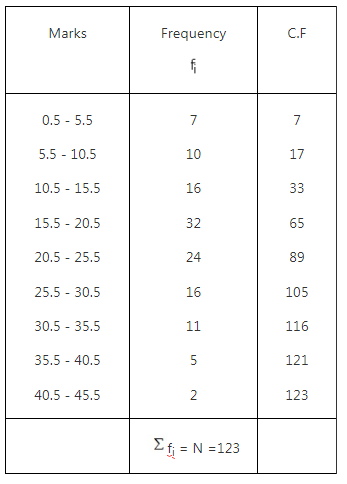N = 123 ⇒ $$\frac { N }{ 2 } =\frac { 123 }{ 2 } =61\cdot 5$$
The cumulative frequency just greater than 61.5 is 65.
The corresponding median class is 15.5 – 20.5.
Then the median class is 15.5 – 20.5
l = 15.5, h = 5, f = 32, c = C.F. preceding median class = 33Hence, Median = 19.95.

Question 14.
Solution:The cumulative frequency just greater than 100 is 116 and the corresponding class is 50 – 60.
Thus the median class is 50 – 60
l = 50, h = 10, f = 24, c = C.F. preceding median class = 92, N/2 = 100Hence, Median = 53.33.

We hope the RS Aggarwal Solutions Class 10 Chapter 9 Mean, Median, Mode of Grouped Data Ex 9b help you. If you have any query regarding RS Aggarwal Solutions Class 10 Chapter 9 Mean, Median, Mode of Grouped Data Ex 9b, drop a comment below and we will get back to you at the earliest.